# 15 Data Visualization

## 15.1 Publication Graphics

### 15.1.1 Learning Objectives

In this lesson, you will learn:

• The basics of the `ggplot2` package to create static plots
• How to use `ggplot2`’s theming abilities to create publication-grade graphics

### 15.1.2 Overview

ggplot2 is a popular package for visualizing data in R. From the home page:

ggplot2 is a system for declaratively creating graphics, based on The Grammar of Graphics. You provide the data, tell ggplot2 how to map variables to aesthetics, what graphical primitives to use, and it takes care of the details. It’s been around for years and has pretty good documentation and tons of example code around the web (like on StackOverflow). This lesson will introduce you to the basic components of working with ggplot2.

#### ggplot vs base vs lattice vs XYZ…

R provides many ways to get your data into a plot. Three common ones are,

• “base graphics” (`plot()`, `hist()`, etc`)
• lattice
• ggplot2

All of them work! I use base graphics for simple, quick and dirty plots. I use ggplot2 for most everything else. ggplot2 excels at making complicated plots easy and easy plots simple enough.

#### Setup

Open a new RMarkdown document and remove the filler text.

First, let’s load the packages we’ll need:

``````  library(dplyr)
library(tidyr)
library(ggplot2)
library(viridis)
library(scales)``````

Let’s get set up to where we were at the end of the data tidying lesson. In that lesson, we walked through this chunk of code to read in our survey data, tidy it up, and join it to the events data.

``````survey_raw <- read_csv("https://dev.nceas.ucsb.edu/knb/d1/mn/v2/object/urn%3Auuid%3A71cb8d0d-70d5-4752-abcd-e3bcf7f14783", show_col_types = FALSE)

survey_clean <- survey_raw %>%
select(-notes) %>%
mutate(Q1 = if_else(Q1 == "1", "below expectations", Q1)) %>%
mutate(Q2 = tolower(Q2))

events <- read_csv("https://dev.nceas.ucsb.edu/knb/d1/mn/v2/object/urn%3Auuid%3A0a1dd2d8-e8db-4089-a176-1b557d6e2786", show_col_types = FALSE)

survey_joined <- left_join(survey_clean, events, by = "StartDate")``````

### 15.1.3 Static figures using `ggplot2`

Every graphic you make in `ggplot2` will have at least one aesthetic and at least one geom (layer). The aesthetic maps your data to your geometry (layer). Your geometry specifies the type of plot we’re making (point, bar, etc.).

Now, let’s plot our results using `ggplot`. `ggplot` uses a mapping aesthetic (set using `aes()`) and a geometry to create your plot. Additional geometries/aesthetics and theme elements can be added to a `ggplot` object using `+`.

Let’s start by just plotting the answers to question 1. `geom_bar` will automatically count them for us.

``````ggplot(survey_joined, aes(x = location)) +
geom_bar()``````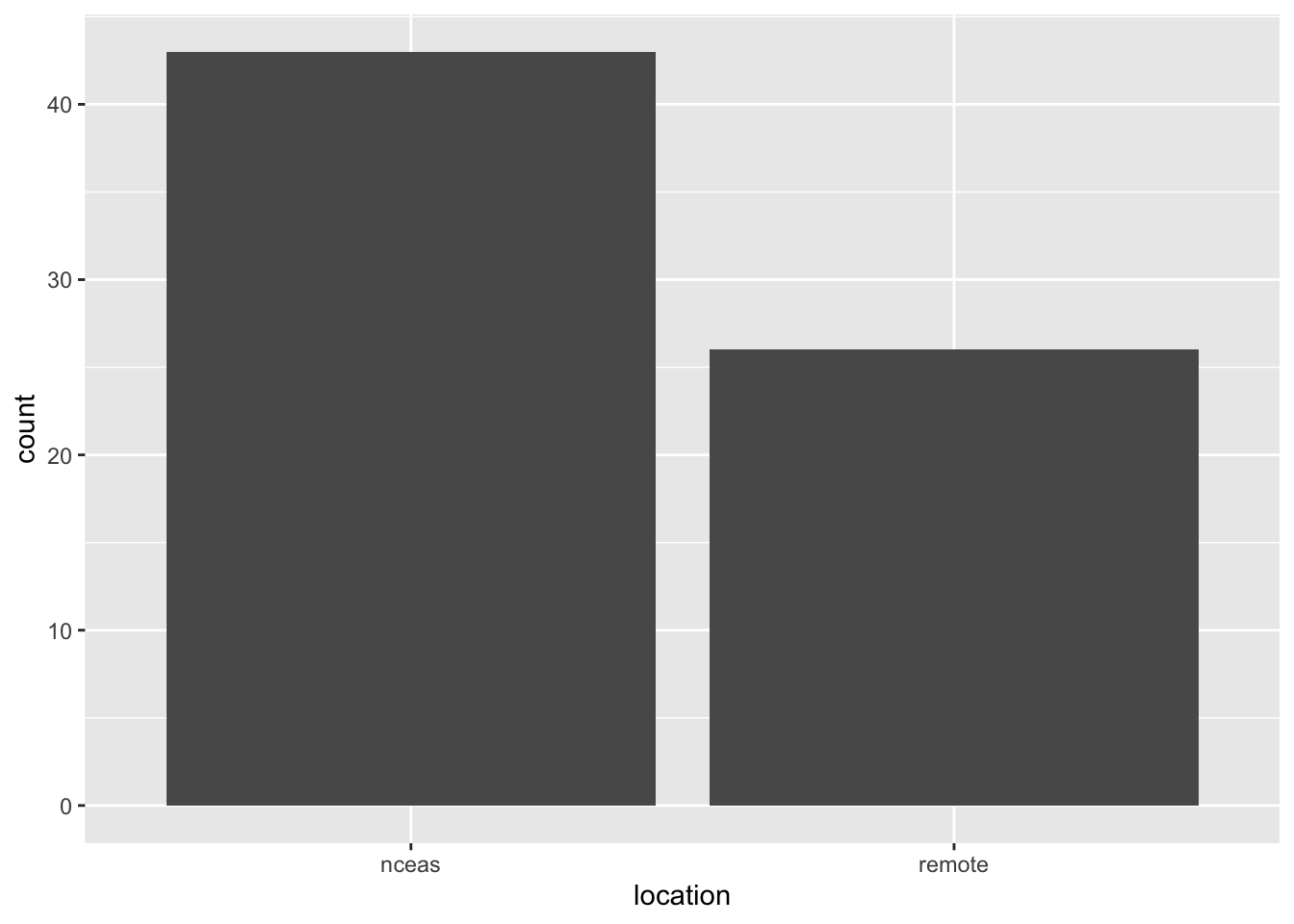What if we want our bars to be blue instad of gray? You might think we could run this:

``````ggplot(survey_joined, aes(x = location, fill = "blue")) +
geom_bar()``````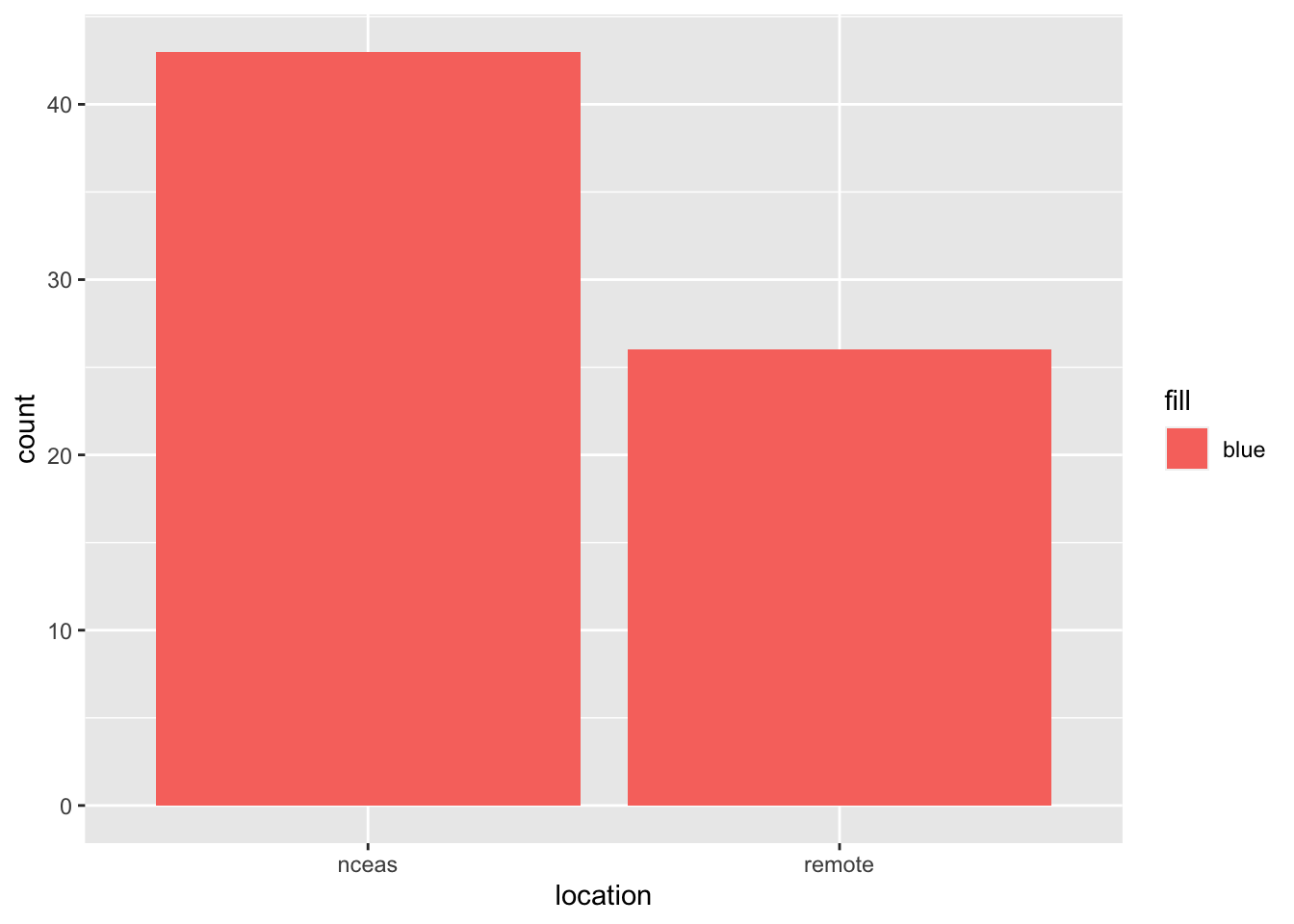Why did that happen?

Notice that we tried to set the fill color of the plot inside the mapping aesthetic call. What we have done, behind the scenes, is create a column filled with the word “blue” in our dataframe, and then mapped it to the `fill` aesthetic, which then chose the default fill color of red.

What we really wanted to do was just change the color of the bars. If we want do do that, we can call the color option in the `geom_bar` function, outside of the mapping aesthetics function call.

``````ggplot(survey_joined, aes(x = location)) +
geom_bar(fill = "blue")``````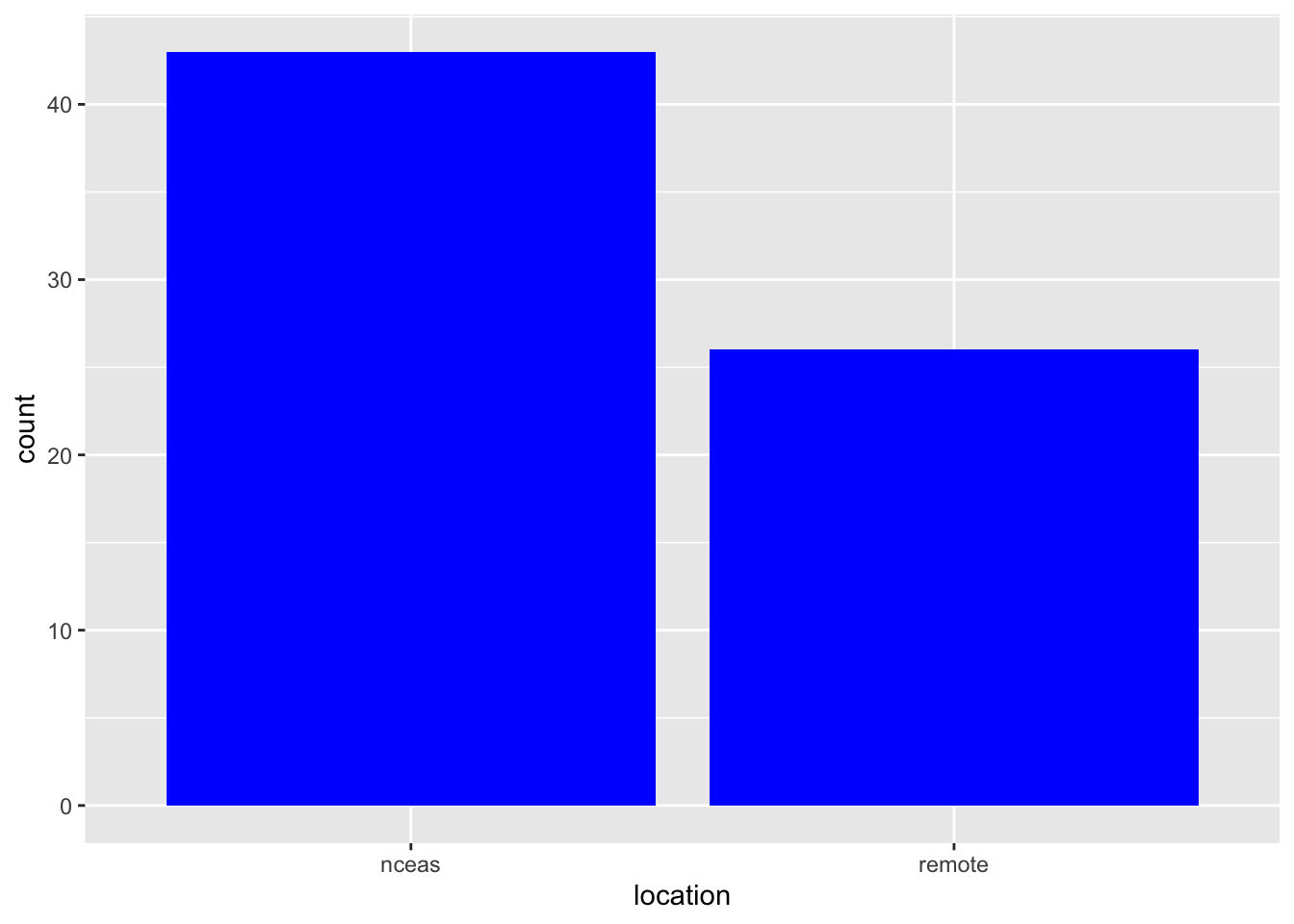What if we did want to map the color of the bars to a variable, such as location of the training.

`ggplot` is really powerful because we can easily get this plot to visualize more aspects of our data.

``````ggplot(survey_joined, aes(x = location, fill = Q1)) +
geom_bar()``````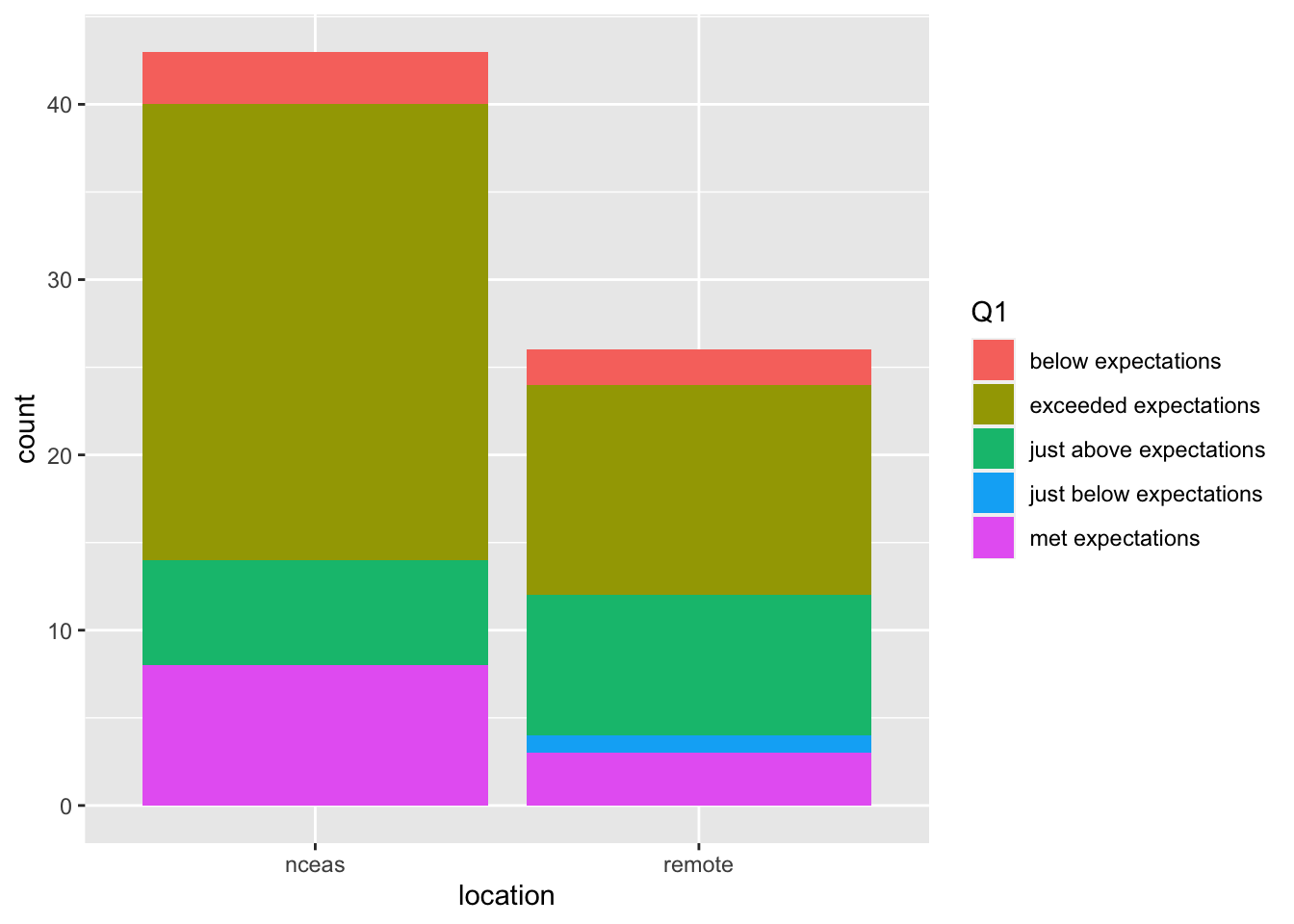If we want to make our bar chart show what percentage of repondents gave each answer, we can switch our bar chart by setting the `position` argument within `geom_bar` to fill.

``````ggplot(survey_joined, aes(x = location, fill = Q1)) +
geom_bar(position = "fill")``````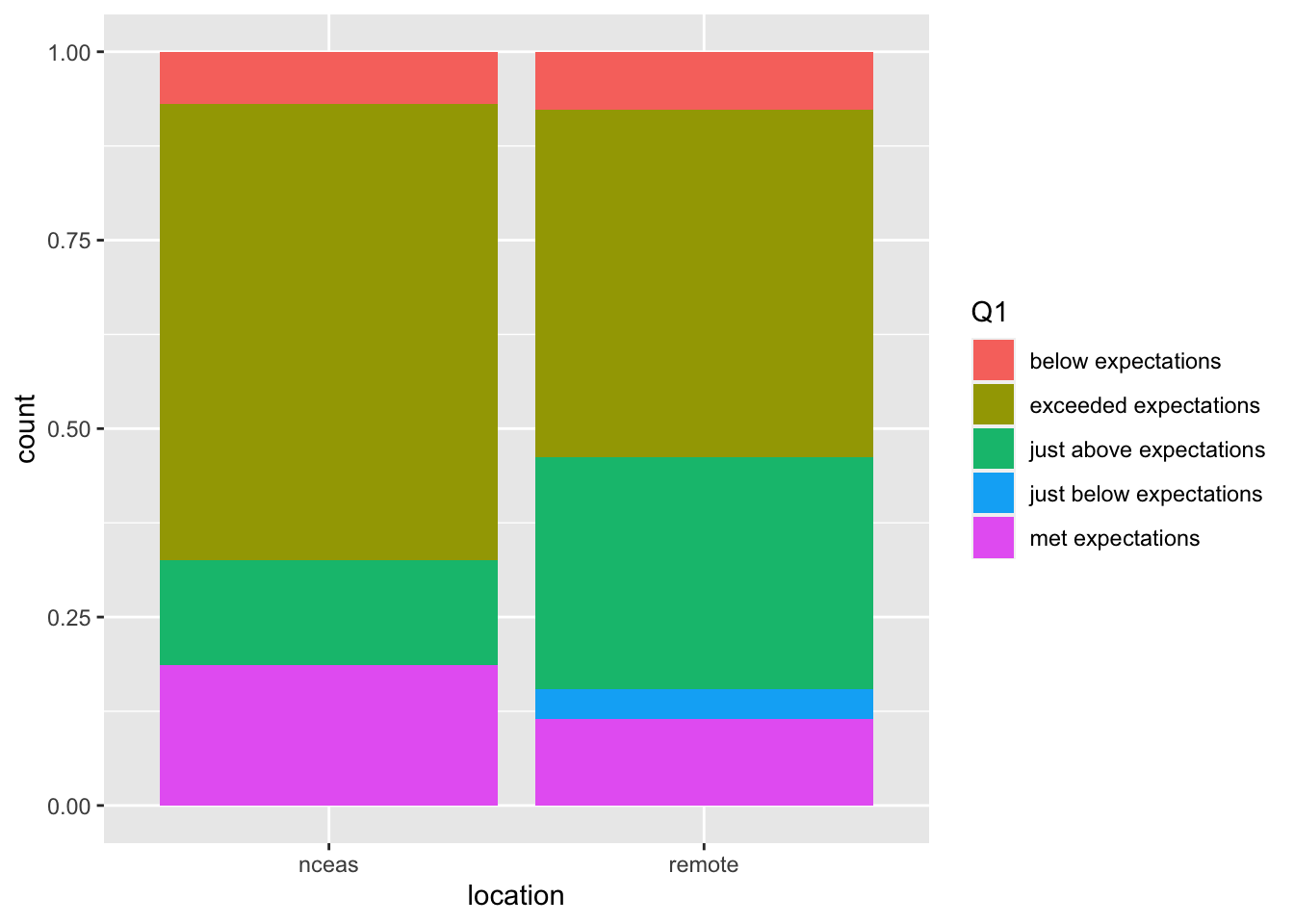#### Setting ggplot themes

Now let’s work on making this plot look a bit nicer. Add labels using the `labs` function, and include a built in theme using `theme_bw()`. There are a wide variety of built in themes in ggplot that help quickly set the look of the plot. Use the RStudio autocomplete `theme_` `<TAB>` to view a list of theme functions.

``````ggplot(survey_joined, aes(x = location, fill = Q1)) +
geom_bar(position = "fill") +
labs(title = "To what degree did the course meet expectations?",
x = "",
y = "Percent of Responses",
fill = "Response") +
theme_bw()``````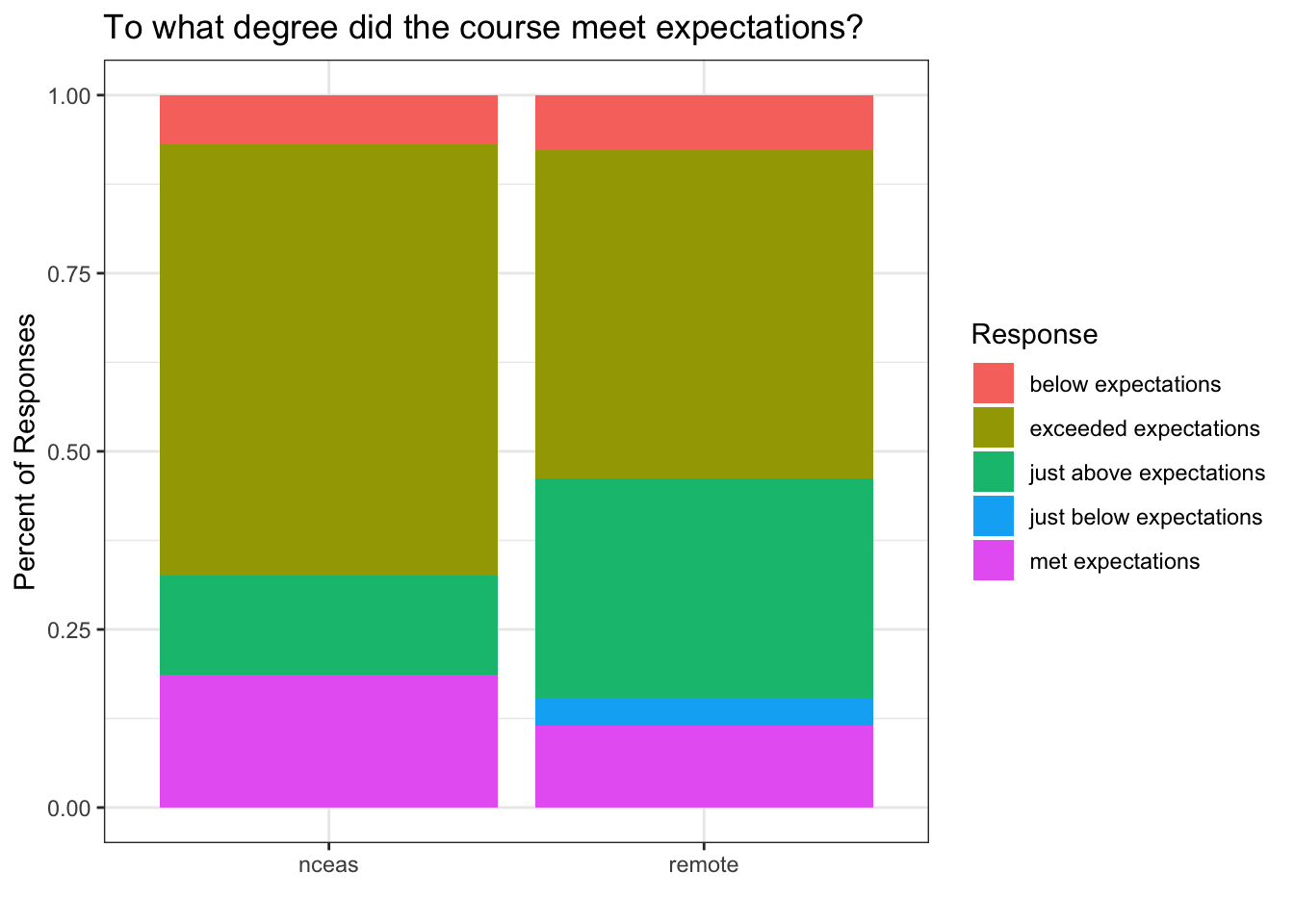You can see that the `theme_bw()` function changed a lot of the aspects of our plot! The background is white, the grid is a different color, etc. There are lots of other built in themes like this that come with the `ggplot2` package.

#### Challenge

Use the RStudio autocomplete, the `ggplot2` documentation, a cheatsheet, or good old google to find other built in themes. Pick out your favorite one and add it to your plot.

The built in theme functions change the default settings for many elements that can also be changed invididually using the`theme()` function. The `theme()` function is a way to further fine-tune the look of your plot. This function takes MANY arguments (just have a look at `?theme`). Luckily there are many great ggplot resources online so we don’t have to remember all of these, just google “ggplot cheatsheet” and find one you like.

Let’s look at an example of a `theme` call, where we change the rotation of the x axis tick labels.

``````ggplot(survey_joined, aes(x = location, fill = Q1)) +
geom_bar(position = "fill") +
labs(title = "To what degree did the course meet expectations?",
x = "",
y = "Percent of Responses",
fill = "Response") +
theme_bw() +
theme(axis.text.x = element_text(angle = 45, vjust = 0.5))``````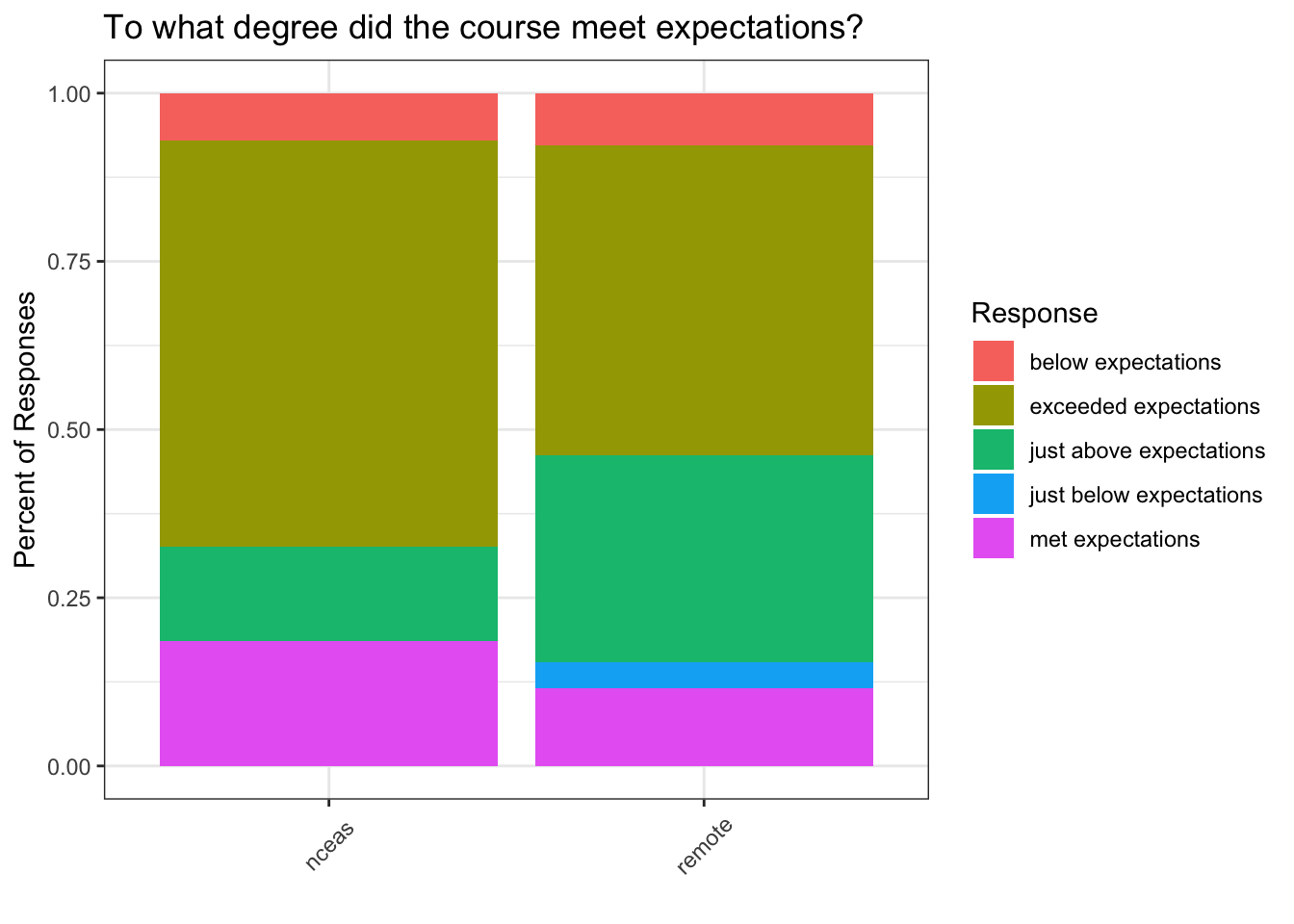Note that the `theme()` call needs to come after any built in themes like `theme_bw()` are used. Otherwise, `theme_bw()` will likely override any theme elements that you changed using `theme()`.

You can also save the result of a series of `theme()` function calls to an object to use on multiple plots. This prevents needing to copy paste the same lines over and over again!

``````my_theme <- theme_bw() +
theme(axis.text.x = element_text(angle = 45, vjust = 0.5))``````
``````ggplot(survey_joined, aes(x = location, fill = Q1)) +
geom_bar(position = "fill") +
labs(title = "To what degree did the course meet expectations?",
x = "",
y = "Percent of Responses",
fill = "Response") +
my_theme``````#### Ordering our responses using `factors`

You might notice that our tick labels don’t appear in a very intuitive order. We would probably prefer to order these so that we have them in order of below, just below, met, just above, and exceeded. We can do this using `factors`. A factor in R is a class of data (like numeric or character) that represents data that contain a fixed and known set of values. Factors are often used in survey data, like the example we are using, as two of our four questions have a set of known values.

We can use the `factor` function to transform our character vector of Q1 responses into a factor, and the `levels` argument to order the levels. To do this, we will combine it with the `mutate` function call.

``````survey_joined <- survey_joined %>%
mutate(Q1 = factor(Q1, levels = c("exceeded expectations",
"just above expectations",
"met expectations",
"just below expectations",
"below expectations")))``````
``````ggplot(survey_joined, aes(x = location, fill = Q1)) +
geom_bar(position = "fill") +
labs(title = "To what degree did the course meet expectations?",
x = "",
y = "Percent of Responses",
fill = "Response") +
my_theme``````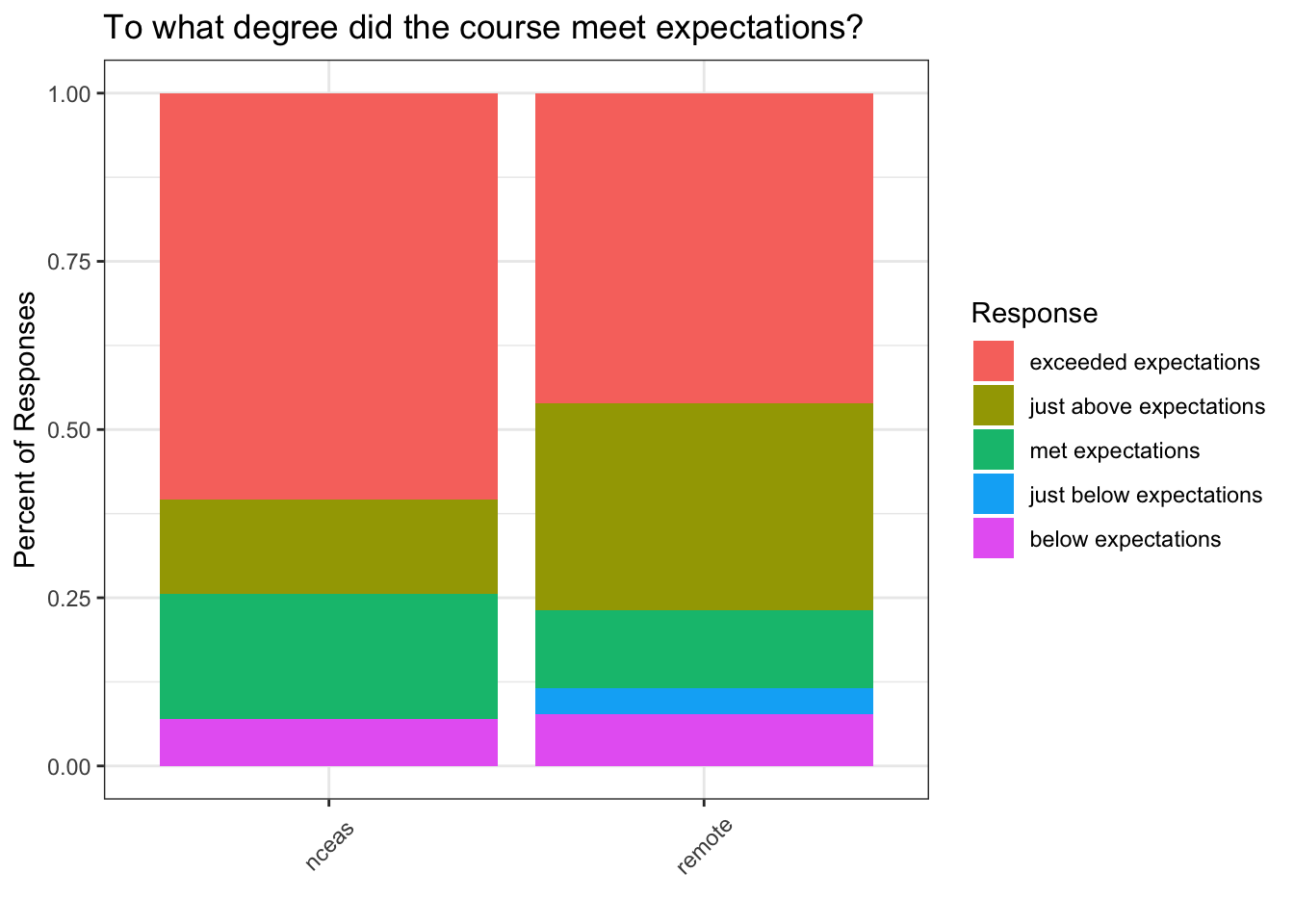One last thing we might want to do is to set a nicer color palette. Here we use `scale_fill_viridis` from the `viridis` package. We can also add `scale_y_continuous` with the `labels = percent` argument to set the y tick labels in percent format.

``````ggplot(survey_joined, aes(x = location, fill = Q1)) +
geom_bar(position = "fill") +
scale_fill_viridis(discrete = TRUE) +
scale_y_continuous(labels = percent) +
labs(title = "To what degree did the course meet expectations?",
x = "",
y = "Percent of Responses",
fill = "Response") +
my_theme``````#### 15.1.3.1 Saving plots

Saving plots using ggplot is easy! The `ggsave` function will save either the last plot you created, or any plot that you have saved to a variable. You can specify what output format you want, size, resolution, etc.

``ggsave("question1.png", width = 3, height = 3, units = "in")``

#### Creating multiple plots

``````survey_long <- survey_joined %>%
pivot_longer(cols = starts_with("Q"), names_to = "id", values_to = "answer") %>%
filter(id %in% c("Q1", "Q2"))``````

What if we wanted to generate a plot for every question with a controlled vocabulary? A fast way to do this uses the function `facet_wrap()`. This function takes a mapping to a variable using the syntax `~variable_name`. The `~` (tilde) is a model operator which tells `facet_wrap` to model each unique value within `variable_name` to a facet in the plot.

The default behavior of facet wrap is to put all facets on the same x and y scale. You can use the `scales` argument to specify whether to allow different scales between facet plots. Here, we free the both scales.

Note that we have had to modify our axes from the previous plots. We now have answer on the y axis and fill as the location, since our answers have different answers.

``````ggplot(survey_long, aes(x = answer, fill = location)) +
geom_bar(position = "dodge") +
scale_fill_viridis(discrete = TRUE) +
labs(x = "", y = "Number of Responses", fill = "Location") +
facet_wrap(~id, scales = "free") +
my_theme``````